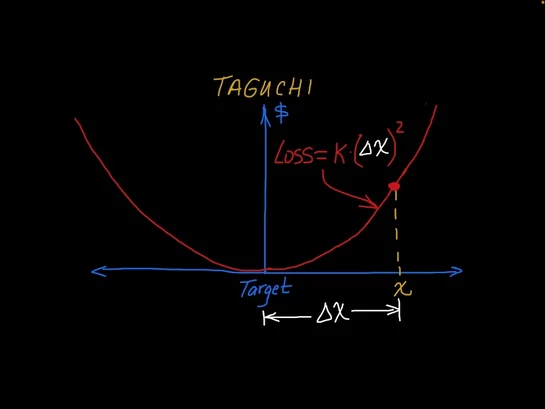# Five (5) things to know about the Taguchi Loss Function:

1. The Taguchi Loss Function (TLF) was developed by Japanese engineer and statistician Genichi Taguchi, who is also known for his contributions to robust design and Taguchi experiments.

2. The TLF states that a deviation of the quality characteristic from its Target value (x - Target) or Δx, incurs a loss proportional to the square of the deviation:k = a constant; sometimes called the Taguchi Loss Coefficient

x = actual value of the quality characteristic

Target = target value of the quality characteristicGraphical illustration of the Taguchi Loss Function and formula

3. For products with an upper/lower spec limit and a Target value in the middle, we can solve for the constant (k) by setting the TLF equal to the product scrap cost at the spec limit (assuming no re-work is possible so that the part needs to be scrapped if outside the spec value). If we do this, the expression for the constant is:The units of k are \$/(unit of Tolerance)^2. The Taguchi Loss can then be expressed as:By inspection, we can see that tightening a tolerance by half increases the loss by 400%! Whereas, an improved design with a tolerance 2X as wide reduces the loss for a given x by 1/4th.

## Example

Say the scrap costs for a given part if it falls outside the tolerance is \$10, the tolerance is 0.010 inches, and the deviation from the target value for a particular part (Δx) is 0.0025 inches. The Taguchi loss for this part is:Decreasing the tolerance to one half its prior value (i.e., 0.005 inches) results in a loss of \$10.00. Increasing the tolerance to 2X its original value (0.020 inches) decreases the loss for the same part to only \$0.625!

- The assumption here, of course, is that the part remains functional for the tolerances above.

4. The TLF makes clear that centering a manufacturing process reduces losses much more than reducing the variation of a process that is way off-center.

5. Taguchi also proposed Loss Functions for Smaller the Better and Larger the Better Quality Characteristics.

• Smaller the Better: when the Target value is zero or a minimum (for example, impurities), Taguchi proposed the following:• Larger the Better: when the Target value is to be as large as possible (e.g. material strength) the loss is given by:If you want to go deeper and find out 3 reasons WHY Taguchi chose a squared loss function, click on this post: 3-Reasons-Taguchi.

105 views

## Related Posts

See All

BLOG

New posts added regularly. To be among the first to know, subscribe.